## MSC (Biotechnology) Final Year Model Papers

#### Top Model Papers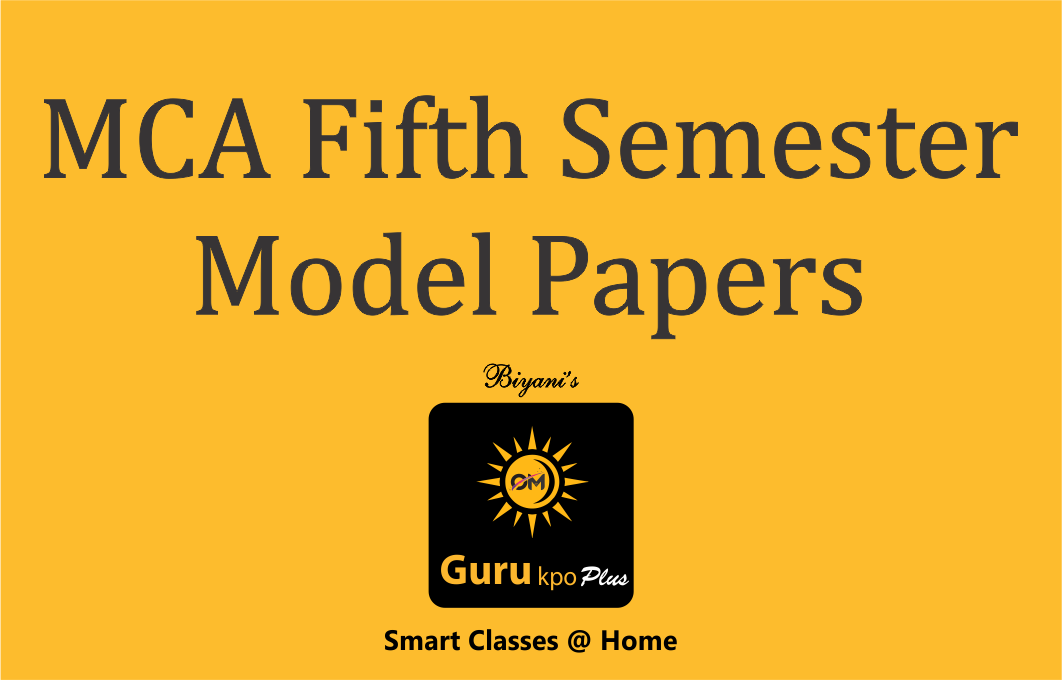#### MCA Fifth Semester Model Papers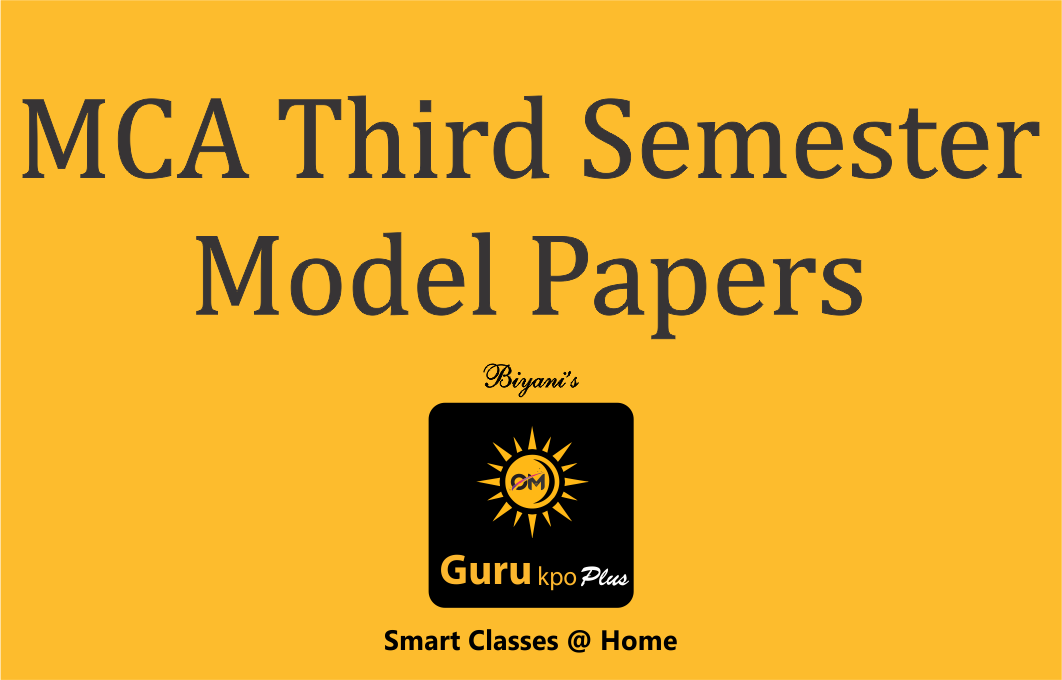#### MCA Third Semester Model Papers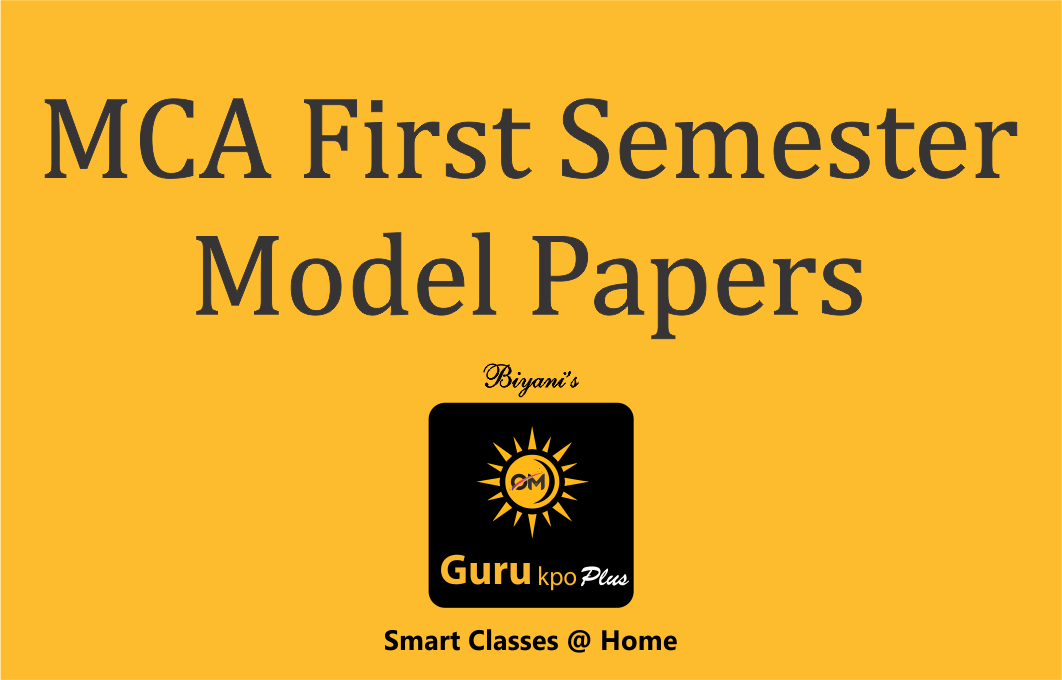#### MCA First Semester Model Papers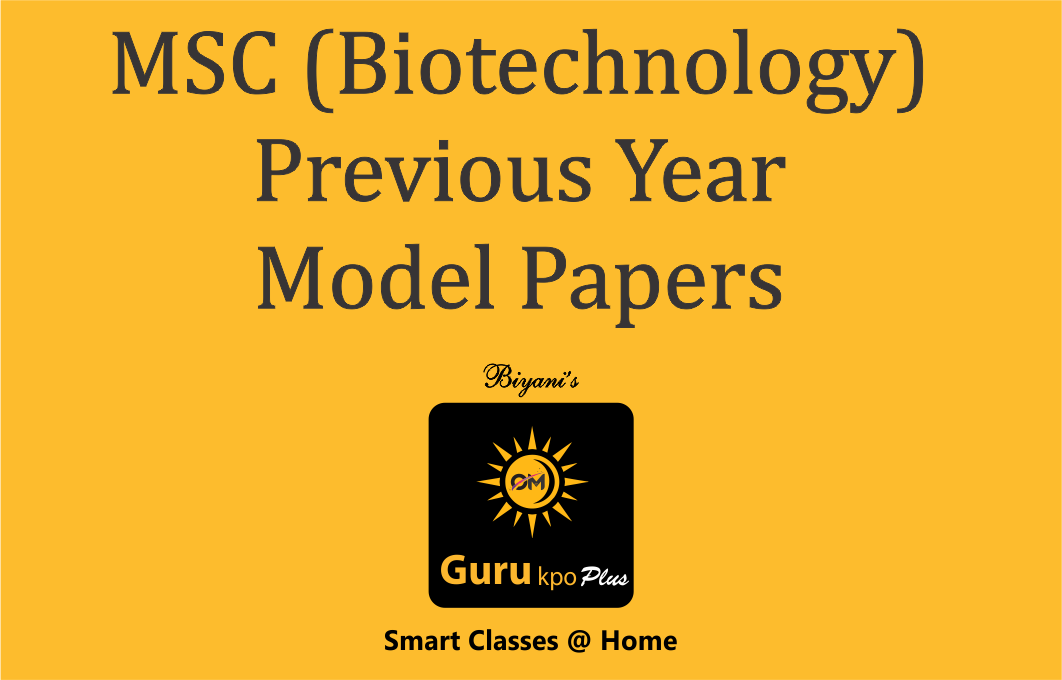#### MSC (Biotechnology) Previous Year Model Papers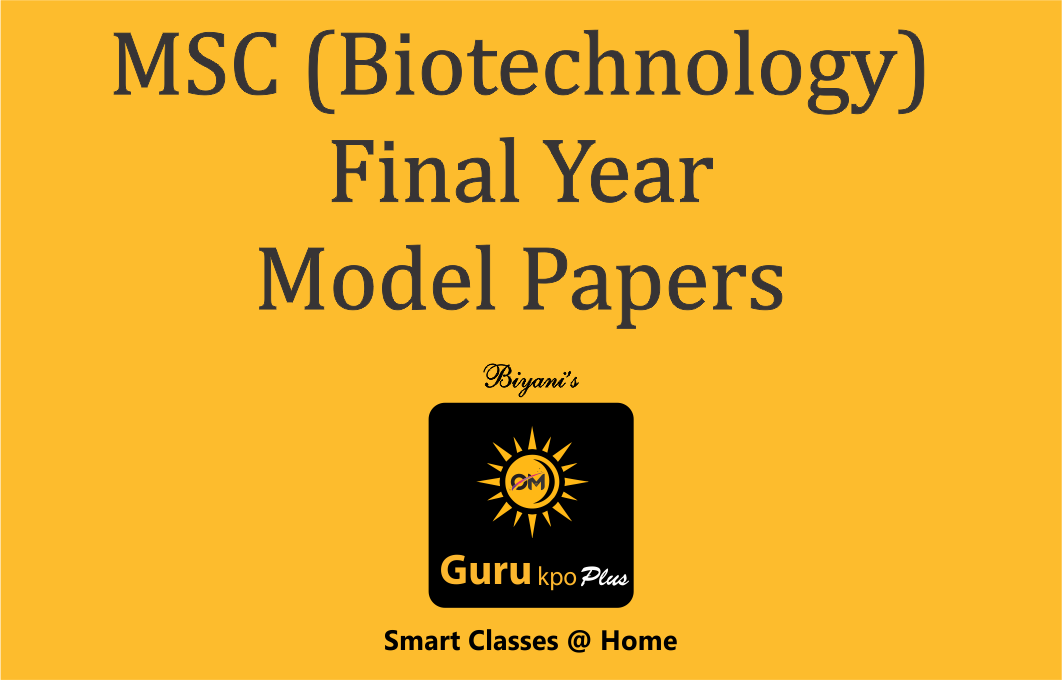#### MSC (Biotechnology) Final Year Model Papers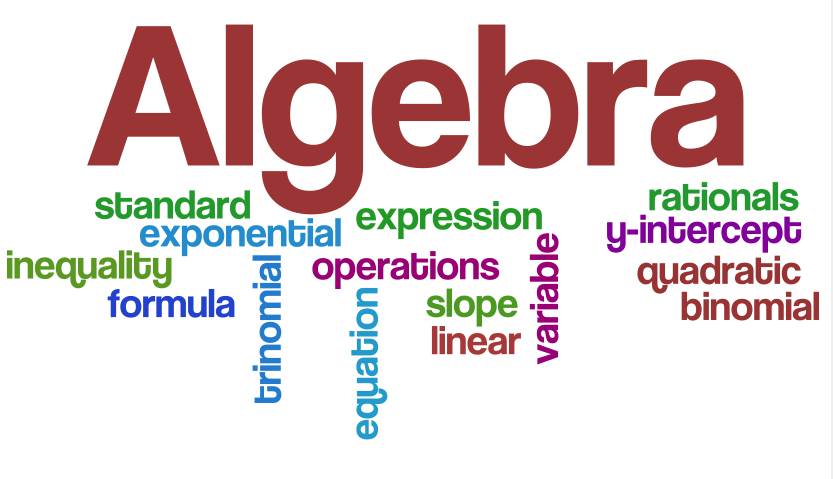Algebra is a branch of mathematics that deals with properties of operations and the structures these operations. Algebra is one of the broad parts of mathematics, together with number theory, geometry and analysis. In its most general form, algebra is the study of mathematical symbols and the rules for manipulating these symbols;
In elementary algebra, those symbols represent quantities without fixed values, known as variables. Just as sentences describe relationships between specific words, in algebra, equations describe relationships between variables. it is a unifying thread of almost all of mathematics. As such, it includes everything from elementary equation solving to the study of abstractions such as groups, rings, and fields.
The basic parts of algebra is elementary algebra, the more abstract parts are called abstract algebra or modern algebra. Elementary algebra is essential for any study of mathematics, science, or engineering, as well as such applications as medicine and economics. Abstract algebra is a major area in advanced mathematics
Simply put, Algebra is about finding the unknown or it is about putting real life problems into equations and then solving them. Unfortunately many textbooks go straight to the rules, procedures and formulas, forgetting that these are real life problems being solved.
A branch of mathematics that substitutes letters for numbers. An algebraic equation represents a scale, what is done on one side of the scale with a number is also done to the other side of the scale. The numbers are the constants. Algebra can include real numbers, complex numbers, matrices, vectors etc.

Application of Algebra:
1. Mathematics is one of the first things we learn in life. Even as a baby we learn to count. Starting from that tiny age you will start to learn how to use building blocks how to count and then move on to drawing objects and figures. All of these things are important preparation to doing algebra.
2. When doing any form of science, whether just a project or a lifetime career choice, we will have to be able to do and understand how to use and apply algebra.
3. Formulas are a part of our lives. Whether we drive a car and need to calculate the distance, or need to work out the volume in a milk container, algebraic formulas are used everyday without realizing it.
4. What about the entering of any data. we use of algebraic expressions and the use of equations will be like a corner stone when working with data entry. When working on the computer with spreadsheets we need algebraic skills to enter, design and plan.
5. How much can we earn on an annual basis with the correct interest rate. How will we know which company gives the best ifwe can’t work out the graphs and understand the percentages. In today’s life a good investment is imperative.
6. we use algebra all the time in real life. It might not happen to involve numbers, but the skills are still there. Say you get home from school one day and you can’t find your key. How would you get into your house? You’d probably do some version of turning the problem around, maybe check the windows to see if you could get in that way, and maybe retrace your steps to see if you dropped your keys somewhere. Eventually, something would work out, and you’d figure out a way to get into your house.
7. Most of us use algebra every day – simple problems that we “do in our heads”. For instance, say you have Rs.20 and you go to the store. The store is having a “buy one and get one at half price” sale. How do you figure out what you can buy? There’s an equation for that. Or, “how tall is that building?” If you know how far away it is, and the height of any one thing you have at hand, there’s an equation for that
8. Like when we are playing games also we use algebra. Pointing from where to start and where to end.